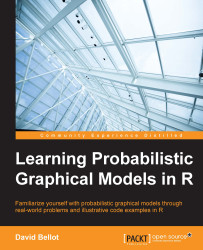•#### Learning Probabilistic Graphical Models in R#### Overview of this book

Probabilistic graphical models (PGM, also known as graphical models) are a marriage between probability theory and graph theory. Generally, PGMs use a graph-based representation. Two branches of graphical representations of distributions are commonly used, namely Bayesian networks and Markov networks. R has many packages to implement graphical models. We’ll start by showing you how to transform a classical statistical model into a modern PGM and then look at how to do exact inference in graphical models. Proceeding, we’ll introduce you to many modern R packages that will help you to perform inference on the models. We will then run a Bayesian linear regression and you’ll see the advantage of going probabilistic when you want to do prediction. Next, you’ll master using R packages and implementing its techniques. Finally, you’ll be presented with machine learning applications that have a direct impact in many fields. Here, we’ll cover clustering and the discovery of hidden information in big data, as well as two important methods, PCA and ICA, to reduce the size of big problems.
Learning Probabilistic Graphical Models in RCreditswww.PacktPub.comPrefaceFree Chapter
Probabilistic ReasoningExact InferenceLearning ParametersBayesian Modeling – Basic ModelsApproximate InferenceBayesian Modeling – Linear ModelsProbabilistic Mixture ModelsAppendixIndex## Learning by inference

In the introduction to this chapter, we saw that learning can be done in a frequentist way by counting data. In most cases, it will be sufficient, but it is also a narrow view of the notion of learning. More generally speaking, learning is the problem of integrating data into the domain knowledge in order to create a new model or improve an existing model. Therefore, learning can be seen as an inference problem, where one updates an existing model toward a better model.

Let's consider a simple problem: modeling the results of tossing a coin. We want to test if the coin is fair or not. Let's call θ the probability that the coin lands on its head. A fair throw would have a probability of 0.5. By tossing the coin several times we want to estimate this probability. Let's say the i-th toss outcome is vi = 1 if the coin shows a head and 0 otherwise. We also assume there is no dependence between each toss, which means observations are i.i.d. And finally, we consider each toss...